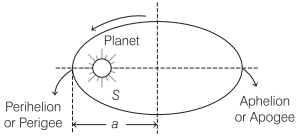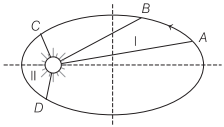# Kepler’s Laws of Planetary Motion | Definition, Formulas – Gravitation

Kepler’s Laws of Planetary Motion Definition:
Kepler’s laws of planetary motion, in astronomy and classical physics, laws describing the motions of the planets in the solar system.

We are giving a detailed and clear sheet on all Physics Notes that are very useful to understand the Basic Physics Concepts.

## Kepler’s Laws of Planetary Motion | Definition, Formulas – Gravitation

Kepler’s Laws of Planetary Motion:

(i) Law of orbit:
Every planet revolves around the sun in an elliptical orbit and sun is at its one focus.(ii) Law of area:
The radius vector drawn from the sun to a planet sweeps out equal areas in equal intervals of time, i.e. the areal velocity of the planet around the sun is constant.
Areal velocity of a planet $$\frac{d \mathbf{A}}{d t}=\frac{L}{2 m}$$ = constantwhere, L = angular momentum and m = mass of the planet.

(iii) Law of period:
The square of the time period of revolution of a planet around the sun is directly proportional to the cube of semi-major axis of its elliptical orbit.
T² ∝ a3 or $$\left(\frac{T_{1}}{T_{2}}\right)^{2}=\left(\frac{a_{1}}{a_{2}}\right)^{3}$$
where, a = semi-major axis of the elliptical orbit.

Gravitation:
Have you ever thought, when we throw a ball above the ground level, why it returns back to the ground. It’s because of gravity. When a ball is thrown above the ground in the opposite direction, a gravitational force acts on it which pulls it downwards and makes it fall. This phenomena is called gravitation.

Learn relation between gravitational field and potential field, Kepler’s law of planetary, weightlessness of objects in absence of gravitation, etc.